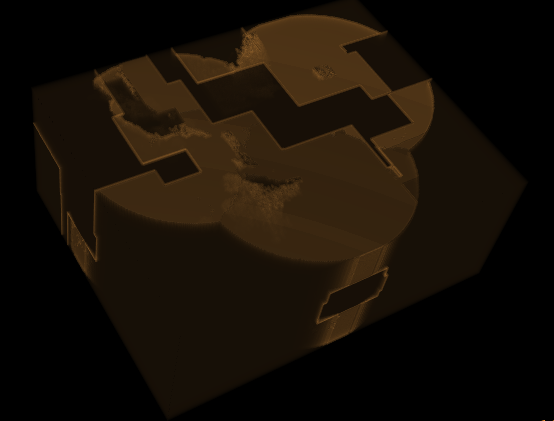# Extract bone

I have few pieces of ideas/code, but I cannot connect them to achieve extraction / removing of a bone from a volume (vtkVolume), with SimpleITK.

I know that I need to make a segmentation on the initial image. And I have tried sitk::SLIC

After that, I have labeled the outcome with LabelIntensityStatisticsImageFilter.

``````Label: {1} -> Mean: {368.290207} Size: {566.464000}
Label: {2} -> Mean: {2526.748027} Size: {1077.480000}
Label: {3} -> Mean: {1322.812512} Size: {2101.808000}
Label: {4} -> Mean: {5.314739} Size: {1655.520000}
Label: {5} -> Mean: {1152.650079} Size: {2021.784000}
Label: {7} -> Mean: {1261.300340} Size: {1532.264000}
Label: {8} -> Mean: {0.115451} Size: {1778.208000}
.....
....
Label: {65} -> Mean: {1173.982044} Size: {2118.912000}
Label: {66} -> Mean: {1282.010115} Size: {1144.464000}
Label: {67} -> Mean: {2.750152} Size: {1684.992000}
Label: {68} -> Mean: {1828.256807} Size: {1617.560000}
Label: {69} -> Mean: {744.446380} Size: {1679.000000}
Label: {70} -> Mean: {2429.636374} Size: {1005.144000}
Label: {71} -> Mean: {0.000000} Size: {1710.064000}
Label: {72} -> Mean: {1037.654082} Size: {1918.584000}
...
...
Label: {205} -> Mean: {661.414440} Size: {2436.288000}
Label: {206} -> Mean: {742.483240} Size: {1631.552000}
Label: {207} -> Mean: {923.733392} Size: {537.688000}
Label: {208} -> Mean: {1080.068644} Size: {410.816000}
Label: {209} -> Mean: {643.469645} Size: {258.800000}
``````

Now, how can I take from those labels the bones only (is CBCT), and use it to remove it from the final sitk::Image ? What are the next filter that should I use these labels to remove bones from the volume ?

Thank you for your time and patience.

Flaviu.

P.S. If I use sitk::ConnectedComponent instead of sitk::SLIC I got only two labels (from a complete CBCT):

``````Label: {1} -> Mean: {1049.634946} Size: {179605.928000}
Label: {2} -> Mean: {12.000000} Size: {0.016000}``````

From my researches I understand that sitk::ChangeLabelImageFilter should be used after sitk::LabelIntensityStatisticsImageFilter. But I didn’t found any example: https://itk.org/ITKExamples/search.html?q=LabelIntensityStatisticsImageFilter

Can you tell me how to use this filter ? Or at least if I am the right way …

P.S. I have tried the following code:

``````	sitk::Image imgTemp(imgOrg);
sitk::Image cc = sitk::ConnectedComponent(imgTemp);
sitk::LabelIntensityStatisticsImageFilter statistics;
statistics.Execute(cc, imgTemp);
std::vector<int64_t>::iterator it;
std::vector<int64_t> labels = statistics.GetLabels();
for (it = labels.begin(); it != labels.end(); ++it)
{
TRACE(_T("Label: {%lu} -> Mean: {%f} Size: {%f}\n"),
*it, statistics.GetMean(*it), statistics.GetPhysicalSize(*it));
}

std::map<double, double> mapChange;
mapChange.insert(std::make_pair(1049.634946, 0));

imgOrg = sitk::ChangeLabel(cc, mapChange);
``````

But I have nothing than a diffuse cube … what I have done wrong ? I feel I am not far from the result, I need a little help …

I come back with a little progress, and with an error:

``````sitk::Image imgTemp(imgOrg);
sitk::Image labels = sitk::SLIC(imgTemp);
sitk::LabelShapeStatisticsImageFilter statistics;
statistics.Execute(labels);

std::vector<int64_t>::iterator it;
std::vector<int64_t> ls = statistics.GetLabels();
std::map<double, double> mapChange;
for (it = ls.begin(); it != ls.end(); ++it)
{
mapChange.insert(std::make_pair(*it, statistics.GetRoundness(*it) < 0.2 ? 0 : 255));
}

sitk::Image change = sitk::ChangeLabel(labels, mapChange);
imgOrg = sitk::LabelMapToBinary(change); // <-- sitk::ERROR: Pixel type: 32-bit unsigned integer is not supported in 3D byclass itk::simple::LabelMapToBinaryImageFilter
``````

but on the last line, imgOrg = sitk::LabelMapToBinary(change);, I got the following error:

`sitk::ERROR: Pixel type: 32-bit unsigned integer is not supported in 3D byclass itk::simple::LabelMapToBinaryImageFilter`

What is the way to overcome this error ?

The `LabelMapToBinaryImageFilter` operates of Images which have a `sitkLabel` pixels type, not regular integers. You can use `NotEqual(change, 0)` to do a similar operation on scalar images.

1 Like

Kindly thank you Bradley, I get rid of that error. But I have to continue to dig in, because after all code from above, even corrected, I got only a diffuse cube, with only small part of opaque brown piece. By now, I only shoot in the darkI have tried sitk::LabelIntensityStatisticsImageFilter instead of sitk::LabelShapeStatisticsImageFilter, with pretty same result. And I guess I got the same result because I still don’t get it what should I put in changeMap from sitk::ChangeLabel As key, and as value.

``````	sitk::Image imgTemp(imgOrg);
sitk::Image cc = sitk::SLIC(imgTemp);
sitk::LabelIntensityStatisticsImageFilter statistics;
statistics.Execute(cc, imgTemp);

std::vector<int64_t> ls = statistics.GetLabels();
std::map<double, double> mapChange;
for (it = ls.begin(); it != ls.end(); ++it)
{
os << *it << "\t" << statistics.GetMean(*it) << "\t" << statistics.GetPhysicalSize(*it)
<< "\t" << statistics.GetRoundness(*it) << std::endl;
mapChange.insert(std::make_pair(*it, statistics.GetPhysicalSize(*it) < 500 ? statistics.GetPhysicalSize(*it) : 0));
}
``````

As map key, is ok *it ?

And as value, *statistics.GetRoundness(it), or *statistics.GetPhysicalSize(it) or *statistics.GetMean(it) ? Or what ? I haven’t found any documentation regarding this …

I think once I’ll understand this, my chance to solve my task will increase … I’ll be very grateful for any clarification here.

I guess I figure out what should put in that mapChange:

``````		if(statistics.GetRoundness(*it) < 0.2)
mapChange.insert(std::make_pair(*it, 0));
``````

and the following filter are:

``````	sitk::Image change = sitk::ChangeLabel(cc, mapChange);
imgOuput = sitk::NotEqual(change, 0);
``````

The problem is that the imgOutput look like that (CBCT):Strange … the filters from output are not the right one … I continue to shoot … in the dark …Instead of “shooting in the dark”, you could go through Segmentation chapter of ITK software guide to get a better start.

2 Likes

Ok, I’ll read it all, even I have read another documentation about this … seem that I haven’t paid enough attention as long I cannot solve my issue.